Four resistances of 100 Ω each are connected in the form of square. Then, the effective resistance along the diagonal points is :

(1) 200 Ω

(2) 400 Ω

(3) 100 Ω

(4) 150 Ω

Concept Questions :-

Combination of resistors
High Yielding Test Series + Question Bank - NEET 2020

Difficulty Level:

Equivalent resistance between the points A and B is (in Ω)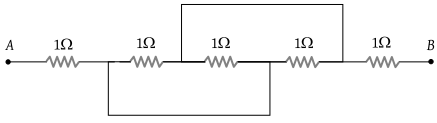(1) $\frac{1}{5}$

(2) $1\frac{1}{4}$

(3) $2\frac{1}{3}$

(4) $3\frac{1}{2}$

Concept Questions :-

Combination of resistors
High Yielding Test Series + Question Bank - NEET 2020

Difficulty Level:

In the circuit shown here, what is the value of the unknown resistor R so that the total resistance of the circuit between points P and Q is also equal to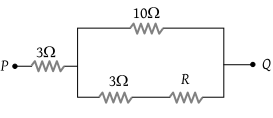(1) 3 ohms

(2) $\sqrt{39}\text{\hspace{0.17em}}ohms$

(3) $\sqrt{69}\text{\hspace{0.17em}}ohms$

(4) 10 ohms

Concept Questions :-

Combination of resistors
High Yielding Test Series + Question Bank - NEET 2020

Difficulty Level:

The resistors of resistances 2 Ω, 4 Ω and 8 Ω are connected in parallel, then the equivalent resistance of the combination will be :

(1) $\frac{8}{7}\Omega$

(2) $\frac{7}{8}\Omega$

(3) $\frac{7}{4}\Omega$

(4) $\frac{4}{9}\Omega$

Concept Questions :-

Combination of resistors
High Yielding Test Series + Question Bank - NEET 2020

Difficulty Level:

The effective resistance of two resistors in parallel is $\frac{12}{7}\text{\hspace{0.17em}\hspace{0.17em}}\Omega$. If one of the resistors is disconnected the resistance becomes 4 Ω. The resistance of the other resistor is :

(1) 4 Ω

(2) 3 Ω

(3) $\frac{12}{7}\text{\hspace{0.17em}\hspace{0.17em}}\Omega$

(4) $\frac{7}{12}\Omega$

Concept Questions :-

Combination of resistors
High Yielding Test Series + Question Bank - NEET 2020

Difficulty Level:

In the circuit, the potential difference across PQ will be nearest to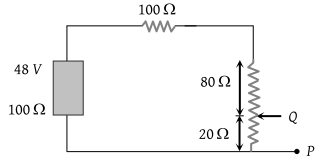(1) 9.6 V

(2) 6.6 V

(3) 4.8 V

(4) 3.2 V

Concept Questions :-

Combination of resistors
High Yielding Test Series + Question Bank - NEET 2020

Difficulty Level:

Three resistors are connected to form the sides of a triangle ABC, the resistance of the sides AB, BC and CA are 40 ohms, 60 ohms and 100 ohms respectively. The effective resistance between the points A and B in ohms will be

(1) 32

(2) 64

(3) 50

(4) 200

Concept Questions :-

Combination of resistors
High Yielding Test Series + Question Bank - NEET 2020

Difficulty Level:

Find the equivalent resistance across AB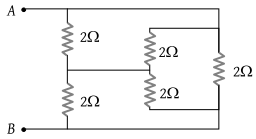(1) 1 Ω

(2) 2 Ω

(3) 3 Ω

(4) 4 Ω

Concept Questions :-

Combination of resistors
High Yielding Test Series + Question Bank - NEET 2020

Difficulty Level:

Two wires of the same dimensions but resistivities ρ1 and ρ2 are connected in series. The equivalent resistivity of the combination is

(1) ρ1 + ρ2

(2) $\frac{{\rho }_{1}+{\rho }_{2}}{2}$

(3) $\sqrt{{\rho }_{1}{\rho }_{2}}$

(4) $2\left({\rho }_{1}+{\rho }_{2}\right)$

Concept Questions :-

Combination of resistors
High Yielding Test Series + Question Bank - NEET 2020

Difficulty Level:

A 3 volt battery with negligible internal resistance is connected in a circuit as shown in the figure. The current I, in the circuit will be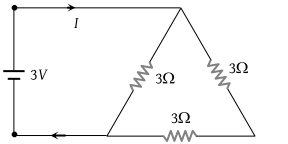(1) 1/3 A

(2) 1 A

(3) 1.5 A

(4) 2 A

Concept Questions :-

Combination of resistors